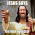## Tuesday, December 16, 2014

### NFL Picks Contest- Week 15 Results and Standings

Here are the Week 15 results and standings in the NFL Picks Contest.

Here are the current standings;

OneAndDone- 27+17+17+34+41+5+31+38+16+24+17+30+32+16+18 = 363 pts.
MOJO-  27+17+17+29+16 +24+32+37+16+34+17+30+32+16+18 = 362 pts.
cokeboy99- 27-(8)+21+34+16+13+20+38+16+51+43+13+32+16+20 = 352 pts.
Captain Crunch- 27+17+17+29+16+5+35+38+24+24-(17)+30+32+34+17 = 328 pts.
Vegas Vic- 27+17+21+29+16+5+32-(3)+20+31+17+29+32+16+17 = 306 pts.

Music City Momma- 13+17+21+29+16-(7)+32+38-(3)+31+17+30+32+17+17 = 300 pts.
mrben09- 27+17+17+29+16+24+31+37-3+24-(15)+30+32+16+18 = 300 pts.
Grange95- (3)+17+21+29+16+24+11+20+16+0+43+13+62+34-(8) = 295 pts.
ggrouchie- 27+17+17+34+41+13+20-(3)+24+24-(15)+30+32+16+18 = 295 pts.
TwoMinuteWarning- (3)+17+37+29+16-(7)+35+37+20+24-(17)+19+32+17+18 = 274 pts.

Neophyte- 27+17-(19)+0+41+24+21+0+24+24+17+14+32+24+28 = 274 pts.
lightning36- 27+17+17+29+16+24+31+24+20+34-(15)+30+32-(31)+18 = 273 pts.
Coach- 27+17+21+29+20+24+20-(3)+16-(7)-(20)+14+11+16+26 = 211 pts.
TeamMiRketti- 27+17+17-(3)+16+24+0-(19)+20+34+0+30+11+0+0 = 174 pts.

Week 15 scores

angerisagift- Atlanta (L 20-27)= (7) pts.
MOJO- Baltimore (W 20-12)= 18 pts.
Vegas Vic- Indianapolis (W 17-10)= 17 pts.
Captain Crunch- Indianapolis (W 17-10)= 17 pts.
OneAndDone- Baltimore (W 20-12)= 18 pts.

mrben09- Baltimore (W 20-12)= 18 pts.
ggrouchie- Baltimore (W 20-12)= 18 pts.
Coach- New Orleans (W 31-15)= 26 pts.
TeamMiRketti-  0 pts.
lightning36- Baltimore (W 20-12)= 18 pts.

Neophyte- Kansas City (W 31-13) = 28 pts.
Music City Momma- Indianapolis (W 17-10)= 17 pts.
TwoMinuteWarning- Baltimore (W 20-12)= 18 pts.
Grange95- Green Bay (L 13-21) = (8) pts.
cokeboy99- Seattle (W 17-7)= 20 pts.

Thanks for playing.

Week 16 picks are due by Saturday at 6pm (CDT). Only Sunday and Monday games are eligible.

Good luck!

1.1.